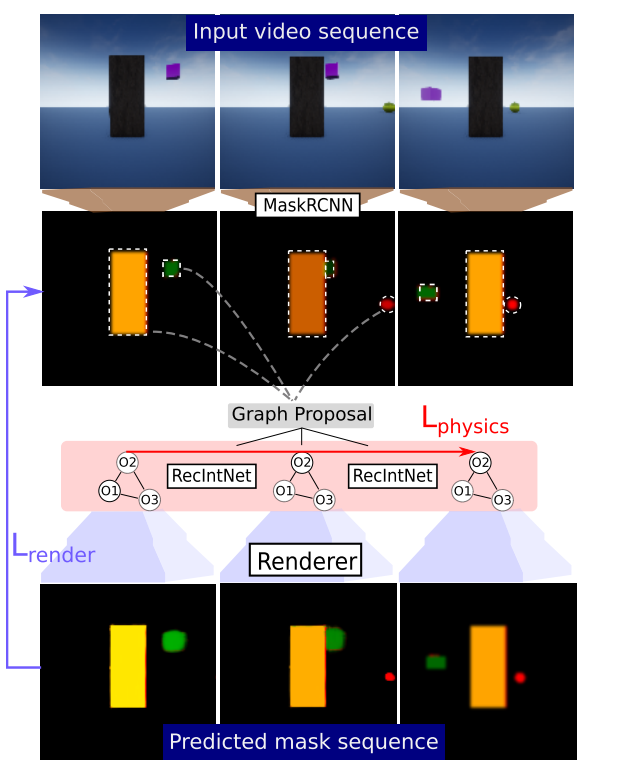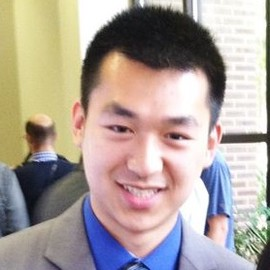# Occlusion resistant learning of intuitive physics from videos

Riochet et al., 2020Source: Riochet et al., 2020

## Summary

• Propose a probabilistic formulation for learning intuitive physics in 3D scenes with significant occlusion
• Object proposals, from a pretrained network or ground-truth segmentations, are linked across frames using a combination of a recurrent interaction network, object-centric dynamics model, and compositional renderer
• Tested on intuitive physics benchmark, synthetic dataset with heavy occlusion, and real videos
• Links: [ website ] [ pdf ]

## Background

• Developing general-purpose methods that can make physical predictions in noisy environments is difficult, especially since objects can be fully occluded by other objects for significant durations
• Video prediction, which operates in pixel space, generally fail to preserve object properties and create blurry outputs, especially as the number of objects increase
• Previous methods that combine object-centric dynamics models with visual encoders have not focused on 3D scenes with significant occlusion
• The proposed method also predicts a plausibility of an observed dynamic scene and infers velocities of objects as latents allowing for trajectory predictions despite occlusion

## Methods

• Event decoding:
• Assign a sequence of underlying object states to a sequence of video frames, $\hat{S} = argmax_S P(S|F,\theta)$
• Can be decomposed into a rendering model, $P(F|S,\theta)$, and a physical model, $P(S|\theta)$
• Simply by:
• expressing state space in 2.5D instead of 3D, bypassing the need to learn inverse projective geometry
• implementing probabilistic models as neural networks
• estimating optimal state, $\hat{S}$, using a combination of a pixel-wise rendering loss and $l_2$ physics loss
• Scene graph proposal gives initial object states, which are linked across time using RecIntNet and a nearest neighbor strategy, then this initial scene interpretation is optimized by minimizing the total loss through both RecIntNet and Renderer on the entire object state sequence
• The inverse of the total loss is used as a plausibility score
• Renderer:
• Predicts a segmentation mask given a list of properties (e.g. $x$ and $y$ position, depth, type, size) for $N$ objects
• Can take a variable number of objects as input and invariant to order of objects
• Object rendering network: reconstructs a segmentation mask and depth map for each object
• Occlusion predictor: composes the $N$ predicted object masks, using the predicted depth maps
• RecIntNet:
• Extends Interaction Networks (Battaglia et al., 2016) in three ways:
• Model 2.5D scenes by adding depth component
• Change to recurrent network, to learn from multiple future frames by “rolling-out” during training
• Directly predict changes in velocity
• Latent object properties unchanged
• Introduce variance in the position predictions, assuming object position follows a multivariate normal distribution, with diagonal covariance
• Weights the physics loss by the estimated noise level

## Results

• Datasets:
• IntPhys: videos of possible and impossible events, split into three blocks where objects may: disappear (O1), change shape (O2), and “teleport” (O3)
• Half of the impossible events occur in plain view, while the other half occurs under full occlusion
• Synthetic dataset with videos of balls of different colors bouncing (on the ground) in a large box
• Five views: Top view (90$^\circ$), top view+occ (moving object occluding 25%), 45$^{\circ}$, 25$^\circ$, and 15$^\circ$
• Real videos from Kinect2 with setup similar to top view of synthetic dataset
• Results are similar to previous SotA on IntPhys for visible scenarios, but much better for occlusion on O1 and O2 datasets
• Does better than simple baselines (Linear, MLP, NoDyn, NoProba) based on $l_2$ error for 5 and 10-frame prediction horizons
• Performace does decrease noticeably as camera angle decreases, i.e. more inter-object occlusion
• Generalization to real videos without any finetuning does okay

## Conclusion

• Requires ground-truth object positions and segmentations, limiting its real world applicaiton
• Using a dynamics model to link observations and incorporating uncertainty are interesting
• Overall the datasets seem to be relatively simple, without any true 3D dynamics